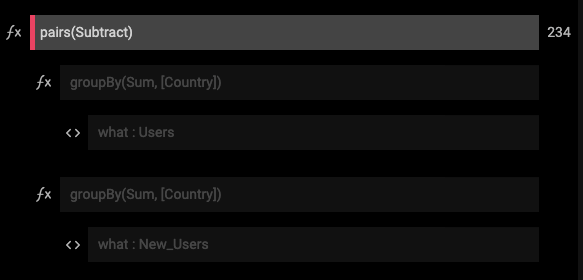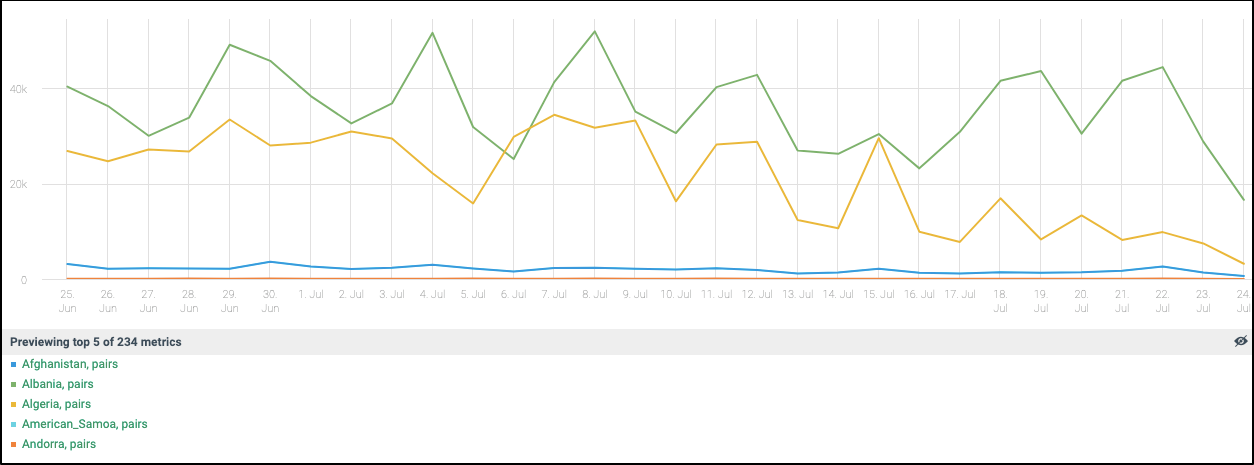Pairs

 Description Divide / multiply / add / subtract pairs of time-series Input Time Series: Multiple (Single or multiple time-series) Parameters Mathematical Operator Divide, multiply, add, subtract Output Grouped Time Series: Multiple Available in Alerts, Composites, Dashboards

The Pairs function provides a simple way to divide / multiply / add / subtract matched pairs of time series.

Usage example

A gaming company is interested in monitoring users adoption rate, by tracking the daily difference between the number of registered users and the number of new users.

The expression below returns the subtraction result of two groups of metrics. The GroupBy function is mandatory here, as the two groups of metrics must be matched in at least one dimension. In this example, we subtract the sum of New_users from a specific country from the sum of Users from the same country. The result of this composite are 234 time series, each one expressing the difference between the matched samples pairs for a specific country:Here is a preview of a few selected metrics. In the selected time range, some countries show stable adoption rates while others exhibit more variable pattern:Note: the RatioPairs function is similar to Pairs, yet limited to the division operator only - often used for generating handy composites such as CPC (Cost Per Click) and CTR (Click Through Ratio).